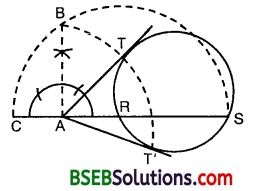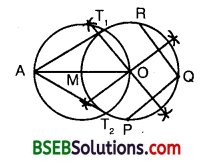# Bihar Board Class 10th Maths Solutions 11 Constructions Ex 11.2

Bihar Board Class 10th Maths Solutions Chapter 11 Constructions Ex 11.2 Textbook Questions and Answers.

## BSEB Bihar Board Class 10th Maths Solutions Chapter 11 Constructions Ex 11.2Question 1.
Draw a circle of radius 6 cm. From a point 10 cm away from its centre, construct the pair of tangents to the circle and measure their lengths.
Solution:
Steps of Construction :
1. Take a point 0 as centre and draw a circle of radius 6 cm.
2. Mark a point P at a distance of 10 cm from the centre O.3. Join OP and bisect it. Let M be its mid-point of OP.
4. With M as centre and MP as radius, draw a circle to intersect the previous circle at Q and R.
5. Join PQ and PR. Then, PQ and PR are the required tangents.
On measurement, we find that PQ = PR = 8 cm.

Justification :
On joining OQ, we find that ∠PQO = 90°, as ∠PQO is the angle in a semi-circle.
∴ PQ ⊥ OQ.
Since OQ is the radius of the given circle, so PQ has to be a tangent to the circle. Similarly, PR is also a tangent to the circle.

Question 2.
Construct a tangent to a circle of radius 4 cm from a point on the concentric circle of radius 6 cm and measure its length. Also, verify the measurement by actual calculation.
Solution:
Steps of Construction :
1. Take a point O and draw two concentric circles of radii 4 cm and 6 cm respectively.
2. Mark a point P on the bigger circle.3. Join OP and bisect it. Let M be its mid-point.
4. With M as centre and MP as radius, draw a circle to intersect the smaller circle at Q and R.
5. Join PQ and PR. Then, PQ and PR are the required tangents.
On measuring, we find that PQ = PR = 4.5 cm (approx.).
Calculation : From A OQP,
OP² = OQ² + PQ²
or 6² = 4² + PQ²
or PQ² = 36 – 16 = 20
So, PQ = $$\sqrt{20}$$cm = 4-47 cm (approx.)
Similarly, PR = 4.47 cm (approx.).

Justification :
On joining OQ, we find that ∠PQO = 90°, as ∠PQO is the angle in a semi-circle.
∴ PQ ⊥ OQ.
Since OQ is the radius of the given circle, so PQ has to be a tangent to the circle. Similarly, PR is also a tangent to the circle.Question 3.
Draw a circle of radius 3 cm. Take two points P and Q on one of its extended diameter each at a distance of 7 cm from its centre. Draw tangents to the circle from these two points P and Q.
Solution:
Steps of Construction :
1. Take a point O, draw a circle of radius 3 cm with this point as centre.
2. Take two points P and Q on one of its extended diameter such that OP = OQ = 7 cm.
3. Bisect OP and OQ. Let their respective mid-points be M1 and M2.
4. With M1 as centre and M1P as radius, draw a circle to intersect the circle at T1 and T2.5. Join PT1 and PT2. Then, PT1 and PT2 are the required tangents.
Similarly, the tangents QTS and QT4 can be obtained.

Justification :
On joining OT4, we find ∠PT10 = 90°, as an angle in a semi-circle.
∴ PT1 ⊥ OT1
Since OT1 is a radius of the given circle, so PT1 has to be a tangent to the circle.
Similarly, PT2, QT3 and QT4 are also tangents to the circle.

Question 4.
Draw a pair of tangents to a circle of radius 5 cm which are inclined to each other at an angle of 60°.
Solution:
Steps of Construction :
1. With O as centre and radius = 5 cm, draw a circle.
2. Draw any diameter AOC.3. Draw a radius OL such that ∠COL = 60° (i.e., the given angle).
4. At L, draw LM ⊥ OL.
5. At A, draw AN ⊥ OA.
6. These two perpendiculars intersect each other at P. Then, PA and PL are the required tangents.

Justification :
Since OA is the radius, so PA has to be a tangent to the circle.
Similarly, PL is the tangent to the circle.
∠APL = 360° – ∠OAP – ∠OLP – ∠AOL
= 360° – 90° – 90° – (180° – 60°)
= 360° – 360° + 60° = 60°
Thus, tangents PA and PL are inclined to each other at an angle of 60°.Question 5.
Draw a line segment AB of length 8 cm. Taking A as centre, draw a circle of radius 4 cm and taking B as centre, draw another circle of radius 3 cm. Construct tangents to each circle from the centre of the other circle.
Solution:
Steps of Construction :
1. Draw a line segment AB = 8 cm.
2. Taking A as centre, draw a circle of radius 4 cm and taking B as centre, draw a circle of radius 3 cm.3. Draw perpendicular bisector of the line segment AB. It intersects the line segment AB at O. Clearly, O is the mid-point of AB. With O as centre, draw a circle of radius OA or OB, intersecting circle with centre B at T4 and T4, circle with centre A at T3 and T4.
4. Join AT1( AT2, BT3 and BT4). Then, these are the required tangents.

Justification :
On joining BT1 we find that ∠BT1A = 90°, as ∠BT1A is the angle in a semi-circle.
∴ AT1 ⊥ BT1
Since BT1 is the radius of the given circle, so AT1 has to be a tangent to the circle.
Similarly, AT2, BT3 and BT4 are the tangents.

Question 6.
Let ABC be a right triangle in which AB = 6 cm, BC = 8 cm and ∠B = 90°. BD is the perpendicular from B on AC. The circle through B, C, D is drawn. Construct the tangents from A to this circle.
Solution:
Steps of Construction :
1. With the given data, draw a A ABC, in which AB = 6 cm, BC = 8 cm and ∠B = 90°.
2. Draw BD ⊥ AC.3. Draw perpendicular bisectors of BC and BD. They meet at a point O.
4. Taking O as centre and OD as radius, draw a circle. This circle passes through B, C and D.
5. Join OA.
6. Draw perpendicular bisector of OA. This perpendicular bisector meets OA at M.
7. Taking M as centre and MA as radius, draw a circle which intersects the circle drawn in step 4 at point B and P.
8. Join AB and AP.
These are the required tangents from A.Question 7.
Draw a circle with the help of a bangle. Take a point outside the circle. Construct the pair of tangents from this point to the circle.
Solution:
Steps of Construction :
1. Draw a circle with the help of a bangle.
2. Draw a secant ARS from an external point A. Produce RA to C such that AR = AC.3. With CS as diameter, draw a semi-circle.
4. At the point A, draw AB ⊥ AS, cutting the semi-circle at B.
5. With A as centre and AB as radius, draw an arc to intersect the given circle, in T and T’. Join AT and AT’. Then, AT and AT’ are the required pair of tangents.

Aliter:1. Mark any three points P, Q and R on the circle.
2. Draw the perpendicular bisectors of PQ and QR to meet at O, the centre of the circle.
3. Join OA and locate its mid-point M.
4. With M as centre and OM as radius, draw a circle to intersect the previous circle at T1 and T2.
5. Join AT1 and AT2, which are the required tangents.